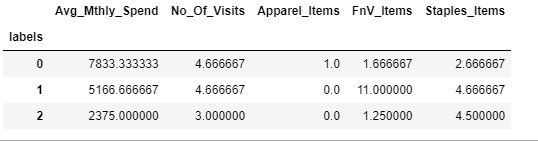top of page
Search

# Agglomerative Hierarchical Clustering Dendogram Assignment Help | What is Hierarchical Clustering?

### Import Necessary Packages

```import pandas as pd
import numpy as np
import matplotlib.pyplot as plt
%matplotlib inline
from scipy.stats import zscore
import seaborn as sns```

```# reading the CSV file into pandas dataframe

Output:```custDataAttr=custData.iloc[:,2:]

Output:```custDataScaled=custDataAttr.apply(zscore)

Output:```#importing seaborn for statistical plots
sns.pairplot(custDataScaled, height=2,aspect=2 , diag_kind='kde')```

Output:```from sklearn.cluster import AgglomerativeClustering
model.fit(custDataScaled)```

Output:

```AgglomerativeClustering(affinity='euclidean', compute_full_tree='auto',
n_clusters=3, pooling_func='deprecated')```

```custDataAttr['labels'] = model.labels_
#custDataAttr.groupby(["labels"]).count()```

Output:```custDataClust = custDataAttr.groupby(['labels'])
custDataClust.mean()```

Output:```from scipy.cluster.hierarchy import cophenet, dendrogram, linkage
from scipy.spatial.distance import pdist  #Pairwise distribution between data points```
```# cophenet index is a measure of the correlation between the distance of points in feature space and distance on dendrogram
# closer it is to 1, the better is the clustering
c, coph_dists = cophenet(Z , pdist(custDataScaled))
c```

Output:

`0.8681149436293064`

```plt.figure(figsize=(10, 5))
plt.title('Agglomerative Hierarchical Clustering Dendogram')
plt.xlabel('sample index')
plt.ylabel('Distance')
dendrogram(Z, leaf_rotation=90.,color_threshold = 40, leaf_font_size=8. )
plt.tight_layout()```

Output:```# cophenet index is a measure of the correlation between the distance of points in feature space and distance on dendrogram
# closer it is to 1, the better is the clustering

c, coph_dists = cophenet(Z , pdist(custDataScaled))
c```

Output:

`0.8606955190809153`

```plt.figure(figsize=(10, 5))
plt.title('Agglomerative Hierarchical Clustering Dendogram')
plt.xlabel('sample index')
plt.ylabel('Distance')
dendrogram(Z, leaf_rotation=90.,color_threshold=90,  leaf_font_size=10. )
plt.tight_layout()```

Output:```# cophenet index is a measure of the correlation between the distance of points in feature space and distance on dendrogram
# closer it is to 1, the better is the clustering

c, coph_dists = cophenet(Z , pdist(custDataScaled))
c```

Output:

`0.8453818941339526`

```plt.figure(figsize=(10, 5))
plt.title('Agglomerative Hierarchical Clustering Dendogram')
plt.xlabel('sample index')
plt.ylabel('Distance')
dendrogram(Z, leaf_rotation=90.,color_threshold=600,  leaf_font_size=10. )
plt.tight_layout()```

Output:If you have any query or need help in any Agglomerative Hierarchical Clustering then send your request at realcode4you@gmail.com and get instant help with an affordable price.